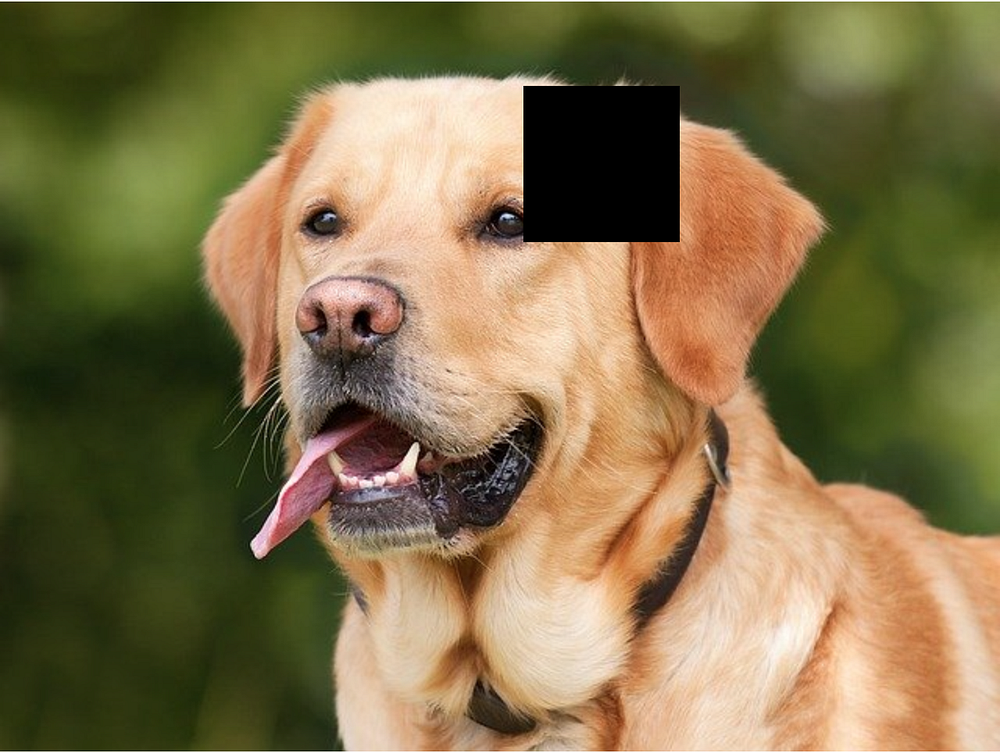# Cutout regularization for CNNs

Source: Deep Learning on Medium# Training the model…

## …with some simple augmentation

The code for ResNet44 can be found in Keras’ documentation here. From there, let’s create an instance of ResNet44:

`model = resnet_v1( input_shape=x_train.shape[1:], depth=44)model.compile( loss='categorical_crossentropy', optimizer=RMSprop(), metrics=['accuracy'])`

I used the learning rate schedule from the documentation of Keras, with four drops over 100 epochs:

`def lr_schedule(epoch): lr = 1e-3if epoch > 85: lr *= 0.5e-3elif epoch > 75: lr *= 1e-3elif epoch > 65: lr *= 1e-2elif epoch > 50: lr *= 1e-1 print(‘Learning rate: ‘, lr)return lrlr_scheduler = LearningRateScheduler(lr_schedule)`

Let us first train a model that uses simple augmentation, using the data augmentation techniques from this paper by He et al., as a reference:

`datagen = ImageDataGenerator( width_shift_range=[-4,4], height_shift_range=[-4,4], horizontal_flip=0.5,  fill_mode=’constant’, cval=0)datagen.fit(x_train)`

This will generate slightly modified images (i.e. slightly translated and potentially flipped around the horizontal axis).

`start = time()hist = model.fit_generator( datagen.flow(x_train, y_train, batch_size=64),  epochs=100,  validation_data=(x_test,y_test), callbacks=[lr_scheduler])duration = time() - startsimple_val_acc = hist.history['val_accuracy']plt.plot([1 - acc for acc in simple_val_acc])plt.title('Validation error for a model using simple augmentation')plt.ylabel('Validation error')plt.xlabel('Epoch')plt.savefig('simple_augmentation_error.png')plt.show()`

## …with cutout regularization

Now, let’s add cutout regularization to the mix. The idea here is to try different values of `M` : this parameter represents how many inputs with cutout we generate from one image in the original training dataset. More specifically, the values we are going to experiment with are 2, 4, 8, 16 and 32.

We’re going to use a generator to feed ResNet44 with batches of images, augmented using our custom cutout function:

`def batch_generator(x, y, epochs, m, batch_size, augment=None):for _ in range(epochs): n = x.shape reorder = np.random.permutation(n) cursor = 0while cursor + batch_size < x.shape: x_batch = x[reorder[cursor:cursor+batch_size]] y_batch = y[reorder[cursor:cursor+batch_size]]if augment != None:yield np.array([augment(xx) for xx in x_batch for rep in range(m)]), np.array([yy for yy in y_batch for rep in range(m)])else:yield x_batch, y_batch cursor += batch_size`

Now, let’s train five models, one for each value of `M`.

`val_acc_cutout = []epochs = 100durations = []for i in [2,4,8,16,32]: model = resnet_v1( input_shape=x_train.shape[1:], depth=44 )  model.compile( loss='categorical_crossentropy', optimizer=RMSprop(), metrics=['accuracy'] )  duration = time() hist = model.fit_generator( batch_generator( x_train, y_train, m=i, batch_size=64, epochs=100,  augment=apply_mask ), epochs=1,  validation_data=(x_test,y_test), steps_per_epoch=np.floor(x_train.shape/64.0), verbose=0, callbacks=[lr_scheduler] ) durations.append(time()-duration) val_acc_cutout.append(hist.history['val_acc'])`

And now, let us take a look at the training history:

`def opp(l):return [1-el for el in l]cutout2_data, cutout4_data, cutout8_data, cutout16_data,  cutout32_data = val_acc_cutoutplt.plot(range(1,101),opp(simple_val_acc),"y-")plt.plot(range(1,101),opp(cutout2_data),"b-")plt.plot(range(1,101),opp(cutout4_data),"c-")plt.plot(range(1,101),opp(cutout8_data),"g-")plt.plot(range(1,101),opp(cutout16_data),"r-")plt.plot(range(1,101),opp(cutout32_data),"m-")plt.legend(["M=0","M=2","M=4","M=8","M=16","M=32"])plt.plot(np.linspace(0,100,10000),[0.06]*10000,"k-")plt.title("Validation error for M instances with cutout generated from each input")plt.xlabel("Number of epochs")plt.ylabel("Validation error")plt.savefig("acc_cutout.png")plt.show()`

We also consider the time each training took (for 100 epochs):

`+-------------------+-----------------------------+| augmentation type | total training time (hours) |+-------------------+-----------------------------+| simple | 1.22 || cutout (M=2) | 1.47 || cutout (M=4) | 2.31 || cutout (M=8) | 3.86 || cutout (M=16) | 6.89 || cutout (M=32) | 13.14 |+-------------------+-----------------------------+`

As we can see, `M=16` and `M=32` both lead to very long training times, while not giving good accuracies. `M=2` leads to a performance comparable to the one obtained without using cutout, and can also be dismissed. However, both models with `M=4` and `M=8` perform better than the original model with no cutout. Since the training is much faster with `M=4` than it is with `M=8`, `M=4` seems to be the best choice here.